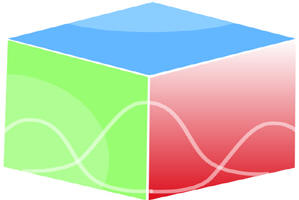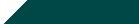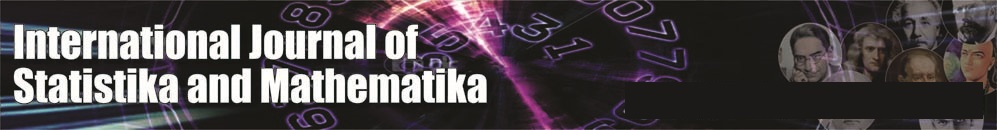``Home| Journals | Statistics Online Expert | About Us | Contact UsUntitled Document

[

Cost-benefit analysis of two-unit cold standby wind turbines system subject to non-Availability of wind and human error

Ashok Kumar Saini

Associate Professor, Department of Mathematics, B. L. J. S. College, Tosham, Bhiwani, Haryana, INDIA.

Email: [email protected]

Research Article

Abstract               Introduction: Humans use wind flow for many purposes: sailing boats, pumping water, grinding mills and also generating electricity. Wind turbines convert the kinetic energy of the moving wind into electricity. The advantages of wind energy is (1) Can be used for both distributed generation or grid interactive power generation using on-shore or off shore technologies (2)Ranges of power producing turbines are available. Micro-turbines are capable of producing 300W to 1MW and large wind turbines have typical size of 35kW-3MW (3) Wind turbine is suitable to install in remote rural area, water pumping and grinding mills. In this paper we have taken failure which is caused due to non-availability of wind energy (NAWE) by wind and open failure mode due to human error. Using semi-Markov technique with regeneration point technique we have evaluated (1) MTSF (2) Availability (3) busy period analysis (4) Expected number of visits by the repairman (5) cost-benefit analysis. Availability of wind energy by wind cannot occur simultaneously in both the units and after failure the unit undergoes repair immediately.

Keywords: Cold Standby Wind Turbines System, Non-Availability of wind energy by wind (NAWE), Human Error, MTSF, Availability, Cost- Benefit Analysis.

INTRODUCTION

Wind Energy for power generation

Wind Energy, like solar is a free energy resource. But is much intermittent than solar. Wind speeds may vary within minutes and affect the power generation and in cases of high speeds- may result in overloading of generator. Energy from the wind can be tapped using turbines. Setting up of these turbines needs little research before being established. Be it a small wind turbine on a house, a commercial wind farm or any offshore installation, all of them, at first, need the Wind Resource to be determined in the area of proposed site. The Wind Resource data is an estimation of average and peak wind speeds at a location based on various meteorological. The next step is to determine access to the transmission lines or nearest control centre where the power generated from the turbines can be conditioned, refined, stored or transmitted. It is also necessary to survey the impact of putting up wind turbines on the community and wildlife in the locality. If sufficient wind resources are found, the developer will secure land leases from property owners, obtain the necessary permits and financing; purchase and install wind turbines. The completed facility is often sold to an independent operator called an independent power producer (IPP) who generates electricity to sell to the local utility, although some utilities own and operate wind farms directly. In this paper we have taken failure caused due to non- availability of wind energy by wind and open failure due to human errors. When the main operative unit fails then cold standby system becomes operative. Availability of wind energy by wind cannot occur simultaneously in both the units and after failure the unit undergoes repair immediately.

Assumptions

• The failure and repair time distributions are all assumed to be negative exponential.
• The repair starts immediately upon failure of units.
• The switches are perfect and instantaneous.
• All random variables are mutually independent.
• When both the units fail, we give priority to operative unit for repair.
• The other failure due to some human errors.
• Repairs are perfect and failure of a unit is detected immediately and perfectly.
• The system is down when the units are non-operative.
• After repair, the units works like a new one.

Symbols for states of the System

λ1, λ2 - failure rate due to non-availability of wind energy by wind(FNAWE) and failure due to human errors(FHE)

G1 (t), G2 (t) – repair time distribution due to type I, II respectively

Superscripts: O, CS, FNAWE, FHE

Operative, Cold Standby, failure due to non-availability of wind energy by wind, failure due to human errors respectively

Subscripts: nawe, awe, he,ur, wr, uR

No availability of wind energy by wind, availability of wind energy by wind, human errors, under repair, waiting for repair, under repair continued from previous state respectively

Up states: 0, 1, 2;

Down states: 3, 4, 5, 6

Regeneration point: 0, 1, 2

STATES OF THE SYSTEM

0(Oawe, CSnawe)

One unit is operative due to availability of wind energy by wind and the other unit is cold standby with non- availability of wind energy by wind in the other unit.

1(FNAWEnawe, ur, Oawe)

The operating unit fails due to FNAWE mode caused by non-availability of wind energy by wind and is under repair immediately of type I and standby becomes operative starts operating with availability of wind energy by wind.

2(FHE nawe, he, ur, Oawe)

The operative unit fails due to human errors (open failure mode) and undergoes repair of type II and the standby unit becomes operative due to availability of wind energy by wind.

3(FNAWEnawe, uR, FHE nawe, he,wr)

The first unit fails due to non-availability of wind energy by wind and under repair is continued from state 1 and the other unit fails due to human error and is waiting for repair of type II and there is non-availability of wind energy by wind.

4(FNAWE nawe, uR, FNAWE nawe, wr)

The one unit fails due to non-availability of wind energy by wind is continues under repair of type I from state 1 and the other unit also fails due to FNAWE is waiting for repair of type I.

5(FHE nawe, he, uR, FNAWE nawe, wr)

The operating unit fails due to human error (open failure mode) and under repair of type I continue from the state 2 and there is non- availability of wind energy by wind and the other unit fails due to FNAWE is waiting for repair of type-II.

6(FHE nase, he, uR, FHE nase, he, wr)

The operative unit fails due to human error and under repair continues from state 2 of type –I and the other unit is also failed due to human error and is waiting for repair of type-I and there is non- availability of wind

energy by wind.

TRANSITION PROBABILITIES

Simple probabilistic considerations yield the following expressions:

p01 + p02 = 1,

p10 + p11(3) + p11(4) = 1,

p20 + p22(5) + p22(6) = 1                                                                                                                                                                       (1)

Figure 1: The State Transition Diagram

And mean sojourn time are

µ0 = E(T) =                                                                                                                                                            (2)

Mean Time to System Failure

Ø0(t) = Q01(t)[s] Ø1(t) + Q02(t)[s] Ø2(t)

Ø1(t) = Q10 (t)[s] Ø0(t) + Q13(t) + Q14(t)

Ø2(t) = Q20 (t)[s] Ø0(t) + Q25(t) + Q26(t)                                                                                                                                    (3-5)

We can regard the failed state as absorbing

Taking Laplace-Stiltjes transform of eq. (3-5) and solving for

ø0*(s)  = N1(s) / D1(s)                                                                                                                                                                      (6)

where

N1(s) = Q01*[ Q13 * (s) + Q14 * (s) ] + Q02*[ Q25 * (s) + Q26 * (s) ]

D1(s) = 1 - Q01* Q10* - Q02* Q20*

Making use of relations (1) and (2) it can be shown that ø0*(0)  =1, which implies that ø0*(t)  is a proper distribution.

MTSF = E[T] = d/ds ø0*(s)   = (D1(0) - N1(0)) / D1 (0)

s=0

= ( +p01  + p02 ) / (1 - p01 p10 - p02 p20 )

where

= 1 + 2,  = 0 + 3 + 4

AVAILABILITY ANALYSIS

Let Mi (t) be the probability of the system having started from state I is up at time t without making any other regenerative state belonging to E. By probabilistic arguments, we have

The value of M0 (t), M1 (t), M2 (t) can be found easily.

The point wise availability Ai (t) have the following recursive relations

A0(t) = M0(t) + q01(t)[c]A1(t) + q02(t)[c]A2(t)

A1(t) = M1(t) + q10(t)[c]A0(t) + q11(3)(t)[c]A1(t)+ q11(4)(t)[c]A1(t),

A2(t) = M2(t) + q20(t)[c]A0(t) + [q22(5)(t)[c]+ q22(6)(t)] [c]A2(t)                                                                                          (7-9)

Taking Laplace Transform of eq. (7-9) and solving for

= N2(s) / D2(s)                                                                                                                                                         (10)

where

N2(s) =  0(s)(1 -  11(3)(s) -  11(4)(s)) (1-  22(5)(s)-  22(6)(s)) + 02(s)  2(s)(1- 11(3)(s) - 11(4)(s))

D2(s) = (1 -  11(3)(s)-  11(4)(s)) { 1 -  22(5)(s) - 22(6)(s) )[1-( 01(s)  10 (s))( 1 -  22(5)(s) - 22(6)(s) ) – (( 01(s)  10 (s))

(1-  11(3)(s)-  11(4)(s))]

A0 =  =  =

Using L’ Hospitals rule, we get

A0 =  =                                                                                                                                      (11)

The expected up time of the system in (0,t] is

(t) =  So that                                                                                                                    (12)

The expected down time of the system in (0,t] is

(t) = t- (t) So that                                                                                                      (13)

The expected busy period of the server when there is failure due to availability of wind energy by wind in (0, t]

R0(t) = q01(t)[c]R1(t) + q02(t)[c]R 2(t)

R1(t) = S1(t) + q01(t)[c]R1 (t) + [q11(3)(t) + q11(4)(t)[c]R1(t),

R2(t) = q20(t)[c]R0(t) + [q22(6)(t)+q22(5)(t)][c]R2(t)                                                                                                                 (14-16)

Taking Laplace Transform of eq. (14-16) and solving for

= N3(s) / D3(s)                                                                                                                                                            (17)

Where

N 3(s) = 01(s)  1(s) and

D 3(s)= (1 -  11(3)(s)-  11(4)(s)) – 01(s) is already defined.

In the long run, R0 =                                                                                                                                                      (18)

The expected period of the system under availability of wind energy by wind in (0, t] is

(t) =  So that

The expected Busy period of the server when there is human error in (0, t]

B0(t) = q01(t)[c]B1(t) + q02(t)[c]B2(t)

B1(t) = q01(t)[c]B1(t) + [q11(3)(t)+ q11(4)(t)] [c]B1(t),

B2(t) = u2(t) + q02(t)[c] B2(t) + [q22(5)(t)+ q22(6)(t)] [c]B2(t)

u2(t) = e- λ1t G2(t)                                                                                                                                                                                               (19- 21)

Taking Laplace Transform of eq. (19-21) and solving for

= N4(s) / D2(s) (22)

where

N4(s) = 02(s)  2(s))

In steady state, B0 =                                                                                                                                                      (23)

The expected busy period of the server for repair in (0,t] is

(t) =  So that                                                                                                                 (24)

The expected number of visits by the repairman for repairing the different units in (0, t]

H0(t) = Q01(t)[s][1+ H1(t)] + Q02(t)[s][1+ H2(t)]

H1(t) = Q10(t)[s]H0(t)] + [Q11(3)(t)+ Q11(4)(t)] [s]H1(t),

H2(t) = Q20(t)[s]H0(t) + [Q22(5)(t) +Q22(6)(t)] [c]H2(t)                                                                                                             (25-27)

Taking Laplace Transform of eq. (25-27) and solving for

= N6(s) / D3(s)                                                                                                                                                           (28)

In the long run, H0 =                                                                                                                                                      (29)

COST BENEFIT ANALYSIS

The cost-benefit function of the system considering mean up-time, expected busy period of the system under availability of wind energy by wind when the units stops automatically, expected busy period of the server for repair of unit, expected number of visits by the repairman for unit failure.

The expected total cost-benefit incurred in (0, t] is

C (t) = Expected total revenue in (0, t] - expected total repair cost in (0, t] due to FNAWE under availability of wind energy by wind when the units automatically stop in (0, t]

• expected total repair cost due to human error for repairing the units in (0, t]
• expected number of visits by the repairman for repairing of the units in (0, t]

The expected total cost per unit time in steady state is

C =  =

= K1A0 - K 2R0 - K 3B0 - K 4H0

Where

K1: revenue per unit up-time,

K2: cost per unit time for which the system is under repair of type- I

K3: cost per unit time for which the system is under repair of type-II

K4: cost per visit by the repairman for units repair.

CONCLUSION

After studying the system, we have analyzed graphically that when the failure due to availability of wind energy by wind rate and failure rate due to human error increases, the MTSF and steady state availability decreases and the cost function decreased as the failure increases.

REFERENCES

1. Barlow, R.E. and Proschan, F., Mathematical theory of Reliability, 1965; John Wiley, New York.
2. Gnedanke, B.V., Belyayar, Yu.K. and Soloyer, A.D., Mathematical Methods of Relability Theory, 1969 ; Academic Press, New York.
3. Dhillon, B.S. and Natesen, J, Stochastic Analysis of outdoor Power Systems in fluctuating environment, Microelectron. Reliab.. 1983; 23, 867-881.
4. Goel, L.R., Sharma, G.C. and Gupta, Rakesh Cost Analysis of a Two-Unit standby system with different weather conditions, Microelectron. Reliab., 1985; 25, 665-659.
5. Goel, L.R., Sharma G.C. and Gupta Parveen, Stochastic Behavior and Profit Analysis of a redundant system with slow switching device, Microelectron Reliab., 1986; 26, 215-219.
6. Cao, Jinhua, Stochastic Behavior of a Man Machine System Operating under Changing Environment subject to a Markov Process with Two States, Microelectron. Reliab., 1989,28, pp. 373-378.
7. Kragt, A., Human Reliability Engineering, IEEE. Trans. Reliab., 1978, R-27, 3, pp.195-201.